Need help? Email help@shout.education

# Bonding in Benzene

## The Kekulé Structure for Benzene, C6H6

### What is the Kekulé structure?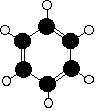Kekulé was the first to suggest a sensible structure for benzene. The carbons are arranged in a hexagon, and he suggested alternating double and single bonds between them. Each carbon atom has a hydrogen attached to it.

This diagram is often simplified by leaving out all the carbon and hydrogen atoms!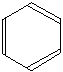In diagrams of this sort, there is a carbon atom at each corner. You have to count the bonds leaving each carbon to work out how many hydrogens there are attached to it.

In this case, each carbon has three bonds leaving it. Because carbon atoms form four bonds, that means you are a bond missing – and that must be attached to a hydrogen atom.

### Problems With the Kekulé Structure

Although the Kekulé structure was a good attempt in its time, there are serious problems with it

#### Problems With the Chemistry

Because of the three double bonds, you might expect benzene to have reactions like ethene – only more so!

Ethene undergoes addition reactions in which one of the two bonds joining the carbon atoms breaks, and the electrons are used to bond with additional atoms.

Benzene rarely does this. Instead, it usually undergoes substitution reactions in which one of the hydrogen atoms is replaced by something new.

#### Problems with the shape

Benzene is a planar molecule (all the atoms lie in one plane), and that would also be true of the Kekulé structure. The problem is that C-C single and double bonds are different lengths.

 C-C 0.154 nm C=C 0.134 nm

Note: "nm" means "nanometre", which is 10-9 metres.

That would mean that the hexagon would be irregular if it had the Kekulé structure, with alternating shorter and longer sides. In real benzene all the bonds are exactly the same – intermediate in length between C-C and C=C at 0.139 nm. Real benzene is a perfectly regular hexagon.

#### Problems with the stability of benzene

Real benzene is a lot more stable than the Kekulé structure would give it credit for. Every time you do a thermochemistry calculation based on the Kekulé structure, you get an answer which is wrong by about 150 kJ mol-1. This is most easily shown using enthalpy changes of hydrogenation.

Help! It doesn't matter whether you've done any thermochemistry sums recently or not. This is all so simple that you could understand it even if you had never done any!

Hydrogenation is the addition of hydrogen to something. If, for example, you hydrogenate ethene you get ethane:

H_2C{=}CH_2 + H_2 \longrightarrow CH_3CH_3

In order to do a fair comparison with benzene (a ring structure) we're going to compare it with cyclohexene. Cyclohexene, C6H10, is a ring of six carbon atoms containing just one C=C.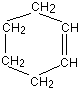Note: If you are a bit shaky on names: cyclohexene: hex means six carbons, cyclo means in a ring, ene means with a C=C bond.

When hydrogen is added to this, cyclohexane, C6H12, is formed. The "CH" groups become CH2 and the double bond is replaced by a single one.

Note: cyclohexane: six carbons in a ring, but the ane ending means NO C=C bond.

The structures of cyclohexene and cyclohexane are usually simplified in the same way that the Kekulé structure for benzene is simplified – by leaving out all the carbons and hydrogens.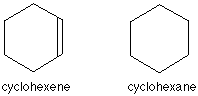In the cyclohexane case, for example, there is a carbon atom at each corner, and enough hydrogens to make the total bonds on each carbon atom up to four. In this case, then, each corner represents CH2.

The hydrogenation equation could be written: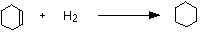The enthalpy change during this reaction is -120 kJ mol-1. In other words, when 1 mole of cyclohexene reacts, 120 kJ of heat energy is evolved.

Help! "Enthalpy change" can be translated as "heat evolved or absorbed". The negative sign shows that heat is evolved.

Where does this heat energy come from? When the reaction happens, bonds are broken (C=C and H-H) and this costs energy. Other bonds have to be made, and this releases energy.

Because the bonds made are stronger than those broken, more energy is released than was used to break the original bonds and so there is a net evolution of heat energy.

If the ring had two double bonds in it initially (cyclohexa-1,3-diene), exactly twice as many bonds would have to be broken and exactly twice as many made. In other words, you would expect the enthalpy change of hydrogenation of cyclohexa-1,3-diene to be exactly twice that of cyclohexene – that is, -240 kJ mol-1.

Note: The name (cyclohexa-1,3-diene) is unimportant. Don't worry about it unless you want to!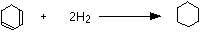In fact, the enthalpy change is -232 kJ mol-1 – which isn't far off what we are predicting.

Note: Thermochemistry sums often throw up discrepancies of this sort of magnitude, and you couldn't be sure whether there was any significance in it.

Applying the same argument to the Kekulé structure for benzene (what might be called cyclohexa-1,3,5-triene), you would expect an enthalpy change of -360 kJ mol-1, because there are exactly three times as many bonds being broken and made as in the cyclohexene case.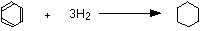In fact what you get is -208 kJ mol-1 – not even within distance of the predicted value!

This is very much easier to see on an enthalpy diagram. Notice that in each case heat energy is released, and in each case the product is the same (cyclohexane). That means that all the reactions "fall down" to the same end point.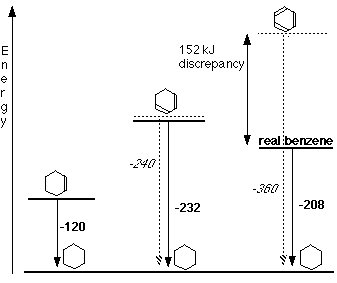Heavy lines, solid arrows and bold numbers represent real changes. Predicted changes are shown by dotted lines and italics.

The most important point to notice is that real benzene is much lower down the diagram than the Kekulé form predicts. The lower down a substance is, the more energetically stable it is.

This means that real benzene is about 150 kJ mol-1 more stable than the Kekulé structure gives it credit for. This increase in stability of benzene is known as the delocalisation energy or resonance energy of benzene. The first term (delocalisation energy) is the more commonly used.

Note: If you look at the diagram closely, you will see that cyclohexa-1,3-diene is also a shade more stable than expected. There is a tiny amount of delocalisation energy involved here as well.

Why is benzene so much more stable than the Kekulé structure suggests? To explain that needs a separate article! Follow the first link below.